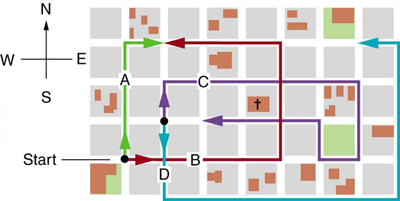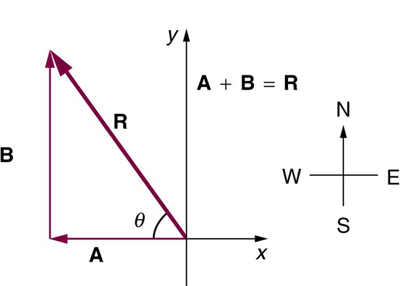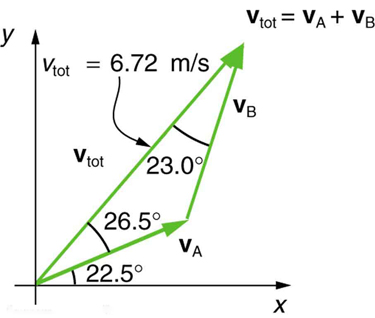# 3.2 Vector addition and subtraction: graphical methods  (Page 5/15)

 Page 5 / 15

## Conceptual questions

Which of the following is a vector: a person’s height, the altitude on Mt. Everest, the age of the Earth, the boiling point of water, the cost of this book, the Earth’s population, the acceleration of gravity?

Give a specific example of a vector, stating its magnitude, units, and direction.

What do vectors and scalars have in common? How do they differ?

Two campers in a national park hike from their cabin to the same spot on a lake, each taking a different path, as illustrated below. The total distance traveled along Path 1 is 7.5 km, and that along Path 2 is 8.2 km. What is the final displacement of each camper?

If an airplane pilot is told to fly 123 km in a straight line to get from San Francisco to Sacramento, explain why he could end up anywhere on the circle shown in [link] . What other information would he need to get to Sacramento?

Suppose you take two steps $\mathbf{\text{A}}$ and $\mathbf{\text{B}}$ (that is, two nonzero displacements). Under what circumstances can you end up at your starting point? More generally, under what circumstances can two nonzero vectors add to give zero? Is the maximum distance you can end up from the starting point $\mathbf{\text{A}}+\mathbf{\text{B}}$ the sum of the lengths of the two steps?

Explain why it is not possible to add a scalar to a vector.

If you take two steps of different sizes, can you end up at your starting point? More generally, can two vectors with different magnitudes ever add to zero? Can three or more?

## Problems&Exercises

Use graphical methods to solve these problems. You may assume data taken from graphs is accurate to three digits.

Find the following for path A in [link] : (a) the total distance traveled, and (b) the magnitude and direction of the displacement from start to finish.The various lines represent paths taken by different people walking in a city. All blocks are 120 m on a side.

(a) $\text{480 m}$

(b) $\text{379 m}$ , $\text{18.4º}$ east of north

Find the following for path B in [link] : (a) the total distance traveled, and (b) the magnitude and direction of the displacement from start to finish.

Find the north and east components of the displacement for the hikers shown in [link] .

north component 3.21 km, east component 3.83 km

Suppose you walk 18.0 m straight west and then 25.0 m straight north. How far are you from your starting point, and what is the compass direction of a line connecting your starting point to your final position? (If you represent the two legs of the walk as vector displacements $\mathbf{\text{A}}$ and $\mathbf{\text{B}}$ , as in [link] , then this problem asks you to find their sum .)The two displacements A size 12{A} {} and B size 12{B} {} add to give a total displacement R size 12{R} {} having magnitude R size 12{R} {} and direction θ size 12{θ} {} .

Suppose you first walk 12.0 m in a direction $\text{20º}$ west of north and then 20.0 m in a direction $\text{40.0º}$ south of west. How far are you from your starting point, and what is the compass direction of a line connecting your starting point to your final position? (If you represent the two legs of the walk as vector displacements $\mathbf{A}$ and $\mathbf{B}$ , as in [link] , then this problem finds their sum .)

$\text{19}\text{.}\text{5 m}$ , $4\text{.}\text{65º}$ south of west

Repeat the problem above, but reverse the order of the two legs of the walk; show that you get the same final result. That is, you first walk leg $\mathbf{B}$ , which is 20.0 m in a direction exactly $\text{40º}$ south of west, and then leg $\mathbf{A}$ , which is 12.0 m in a direction exactly $\text{20º}$ west of north. (This problem shows that $\mathbf{A}+\mathbf{B}=\mathbf{B}+\mathbf{A}$ .)

(a) Repeat the problem two problems prior, but for the second leg you walk 20.0 m in a direction $\text{40.0º}$ north of east (which is equivalent to subtracting $\mathbf{\text{B}}$ from $\mathbf{A}$ —that is, to finding $\mathbf{\text{R}}\prime =\mathbf{\text{A}}-\mathbf{\text{B}}$ ). (b) Repeat the problem two problems prior, but now you first walk 20.0 m in a direction $\text{40.0º}$ south of west and then 12.0 m in a direction $\text{20.0º}$ east of south (which is equivalent to subtracting $\mathbf{\text{A}}$ from $\mathbf{\text{B}}$ —that is, to finding $\mathbf{\text{R}}\prime \prime =\mathbf{\text{B}}-\mathbf{\text{A}}=-\mathbf{\text{R}}\prime$ ). Show that this is the case.

(a) $\text{26}\text{.}\text{6 m}$ , $\text{65}\text{.}\text{1º}$ north of east

(b) $\text{26}\text{.}\text{6 m}$ , $\text{65}\text{.}\text{1º}$ south of west

Show that the order of addition of three vectors does not affect their sum. Show this property by choosing any three vectors $\mathbf{A}$ , $\mathbf{B}$ , and $\mathbf{C}$ , all having different lengths and directions. Find the sum then find their sum when added in a different order and show the result is the same. (There are five other orders in which $\mathbf{A}$ , $\mathbf{B}$ , and $\mathbf{C}$ can be added; choose only one.)

Show that the sum of the vectors discussed in [link] gives the result shown in [link] .

$\text{52}\text{.}\text{9 m}$ , $\text{90}\text{.}\text{1º}$ with respect to the x -axis.

Find the magnitudes of velocities ${v}_{\text{A}}$ and ${v}_{\text{B}}$ in [link]The two velocities v A size 12{v rSub { size 8{A} } } {} and v B size 12{v rSub { size 8{B} } } {} add to give a total v tot size 12{v rSub { size 8{"tot"} } } {} .

Find the components of ${v}_{\text{tot}}$ along the x - and y -axes in [link] .

x -component 4.41 m/s

y -component 5.07 m/s

Find the components of ${v}_{\text{tot}}$ along a set of perpendicular axes rotated $\text{30º}$ counterclockwise relative to those in [link] .

does the force in a system result in the energy transfer?
full meaning of GPS system
how to prove that Newton's law of universal gravitation F = GmM ______ R²
sir dose it apply to the human system
prove that the centrimental force Fc= M1V² _________ r
prove that centripetal force Fc = MV² ______ r
Kaka
how lesers can transmit information
griffts bridge derivative
below me
please explain; when a glass rod is rubbed with silk, it becomes positive and the silk becomes negative- yet both attracts dust. does dust have third types of charge that is attracted to both positive and negative
what is a conductor
Timothy
hello
Timothy
below me
why below you
Timothy
no....I said below me ...... nothing below .....ok?
dust particles contains both positive and negative charge particles
Mbutene
corona charge can verify
Stephen
when pressure increases the temperature remain what?
remains the temperature
betuel
what is frequency
define precision briefly
CT scanners do not detect details smaller than about 0.5 mm. Is this limitation due to the wavelength of x rays? Explain.
hope this helps
what's critical angle
The Critical Angle Derivation So the critical angle is defined as the angle of incidence that provides an angle of refraction of 90-degrees. Make particular note that the critical angle is an angle of incidence value. For the water-air boundary, the critical angle is 48.6-degrees.
okay whatever
Chidalu
pls who can give the definition of relative density?
Temiloluwa
the ratio of the density of a substance to the density of a standard, usually water for a liquid or solid, and air for a gas.
Chidalu
What is momentum
mass ×velocity
Chidalu
it is the product of mass ×velocity of an object
Chidalu
how do I highlight a sentence]p? I select the sentence but get options like copy or web search but no highlight. tks. src
then you can edit your work anyway you wantByByBy Steve GibbsBy Madison ChristianBy RhodesBy Richley CrapoBy Robert MurphyBy Wey HeyBy OpenStaxByBy Janet ForresterBy Brooke Delaney